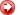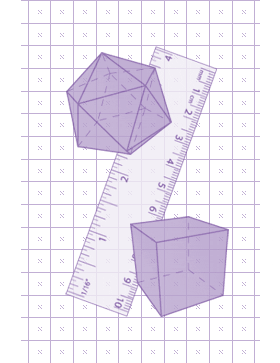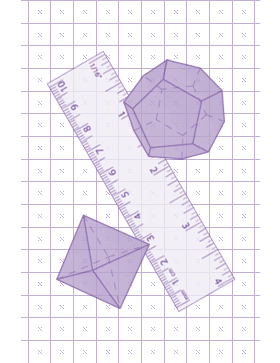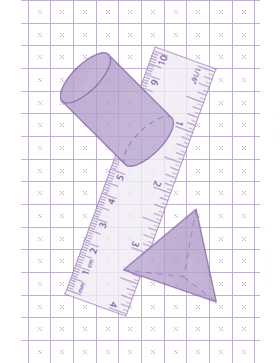Introduction | Goals | How to Use This Site | Credits## Introduction

Geometry 3-D Shapes is an interactive Web site where students can learn about three-dimensional shapes, calculate surface area and volume, and discover some of the mathematical properties of shapes.

Students will analyze the attributes and properties of basic three-dimensional shapes, such as pyramids, prisms, and cylinders. They will develop mathematical arguments about the relationships they discover, such as Euler's theorem, which describes the relationship between the number of faces, vertices, and edges of any polyhedron. Students will distinguish between surface area and volume and learn how to calculate both. Students will also conduct an in-depth exploration of a special group of polyhedra--the Platonic solids.## Goals

The goal of this site is to provide educators and students with the strategies, content, and activities that can enhance and improve students' understanding of geometry.

According to the National Council of Teachers of Mathematics, grades 6-8 students should be able to:
• Precisely describe, classify, and understand relationships among types of two- and three-dimensional objects using their defining properties;

• Use two-dimensional representations of three-dimensional objects to visualize and solve problems such as those involving surface area and volume; and,

• Use geometric models to represent and explain numerical and algebraic relationships (NCTM, 2000).

With these expectations in mind, the specific goals of Geometry 3-D Shapes are for students to be able to:
• Learn the geometric vocabulary of three-dimensional shapes.
The defining properties of three-dimensional objects are their faces, edges, and vertices. In the beginning of this unit, these terms are defined. Throughout the unit, students will be exposed to these terms as they are continuously used and applied in various contexts. At the end of the unit, students will investigate the properties of a special group of polyhedra known as the Platonic Solids.

• Create and use two-dimensional representations of three dimensional shapes.
The net of a three-dimensional object is useful for understanding its properties. For instance, the net of a cylinder emphasizes that it consists of two circles and a rectangle. Students will use nets to analyze figures as well as to calculate surface area.

• Understand the difference between surface area and volume and learn how to calculate each.
When NCTM released the Curriculum Focal Points, a document that details the most important mathematics that students should learn in each grade between kindergarten and 8th grade, they suggested that middle-grades students should "develop an understanding of and use formulas to determine surface areas and volumes of three-dimensional shapes." Whenever possible, students should develop formulas and procedures meaningfully through investigation instead of memorization (NCTM, 2006). Using nets, students will calculate the surface area of prisms, pyramids, cylinders, and other three-dimensional shapes, and using unit cubes, they will determine their volume. These explorations will lead to discovery of the formulas for surface area and volume of many three-dimensional shapes.

• Consider connections between geometry and algebra.
Through geometric investigation, students will discover and apply Euler's Formula, an algebraic equation that describes the relationship between the number of faces, vertices, and edges of a polyhedron.

In keeping with the expected knowledge of students in grades 6-8, Geometry 3-D Shapes will help assess the skills needed to be successful in a geometry class. By using the whole testing approach with printable scorecard, teachers will have a better understanding of each student's strengths and weaknesses.

With the use of randomized multiple choice questions, problem solving exercises, and fill-in-the blank questions; students will have numerous opportunities to illustrate what they know about geometry. Randomized questions allow students to retry exercises with new problems every time. A final assessment test allows teachers to quickly discover which area a student needs to review.## How to Use This Site

Geometry 3-D Shapes consists of four sessions and an assessment. Each session begins with a brief introduction, which provides an overview of the topic. In this introduction, there may be terms that are new to students. A major objective is to give students numerous opportunities to learn the geometric vocabulary of three-dimensional shapes. A glossary is included to provide definitions of key terms.

Students should read the introduction for each section carefully, as it gives a basic overview of concepts and defines important terms. The words that will be used throughout the section are presented here, and each new term is linked to the Glossary. Use the Glossary to look up definitions of terms that are unfamiliar.

Following the introduction, there is an interactive component that allows students to explore three-dimensional geometric figures through animations. Many of the animations simulate a flat sheet of paper (the "net" of the shape) as it comes to life and folds into a three-dimensional object. Use the animations to answer questions and solve related problems. If necessary, a Play/Pause button can be used to suspend an animation or to play it again. They can be played as many times as necessary until students understand what is happening.

The Geometry 3D Shapes lesson can be incorporated into a larger unit about three-dimensional objects and concepts. It provides a solid foundation of the terminology associated with three-dimensional shapes, and it provides a good introduction to some advanced concepts, such as Euler's formula and Platonic solids. Perhaps most importantly, it allows students to develop formulas for surface area and volume on their own and then apply those formulas to a series of practice problems. Moreover, students develop a solid understanding of these ideas through the use of nets to develop the concept.

### Technical Requirements

1. A calculator

2. Browser using Internet Explorer 5 (and higher) and Mozilla 5 (and higher). Best results will be with using latest browser versions

3. Flash player 7 minimum requirement

4. Javascript in browser is turned on## Credits

### Content Production

Patrick Vennebush, Writer
Vennebush is the Online Projects Manager for National Council of Teachers of Mathematics (NCTM) whose primary responsibility is for the Illuminations web site (illuminations.nctm.org). Patrick also serves as Mathematics Leader for Interactive School Mathematics, a middle grades online curriculum being produced by Math Resources, Inc. This project involved writing an entirely computer-based curriculum for middle school that provided a gazillion applets to help the kids learn math interactively. Also a writer for Annenberg's Insights into Algebra as well as a former Associate Director at PBS TeacherLine, Vennebush has created many online courses for K-12 teachers to use as professional development.

Leslie Kriesel, Lisa Weinberger, Copy Editors

Anthony Chapman, Director of Interactive and Broadband
Mikki Monkolchayut, Producer
Dave Hirmes, Flash Action Script Programmer
Marcel Ray, Flash Action Script Programmer
Brian Santalone, HTML Implementation
Yelena Drozd, Flash 3-D Animator
Ying Zhou-Hudson, Graphics ProductionNext: Explore this interactive